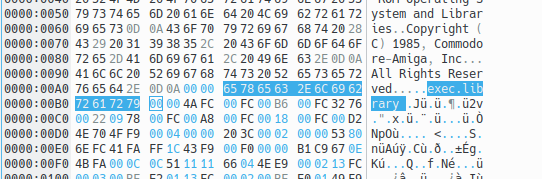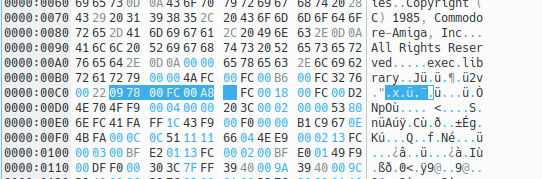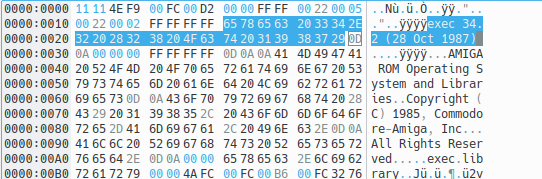By Leonardo Giordani Published on Last update on

# Exec and the vector table¶

The Exec library is the base library of the Amiga system. This library is loaded in memory at boot time, and it is always open and available. Once loaded, it has the same structure of any other library, that is a prefix containing the jump table in reverse order, then the actual code.

The trick here is that Exec is the library used to load in memory other libraries, so the function that creates the structure in memory of a given library is contained here. To install Exec in memory, thus, we need to use a function which is part of the library itself.

This is one of the powers of the Assembly language. The property of treating the code as if it was pure data is called homoiconicity, and is something that can be rarely found in other languages. Lisp is a good example of a higher level homoiconic language.

Back to our vector table, we have to find a way to use the Exec library to install in memory the Exec library itself. The concept is not that complex, actually. The pseudocode is something like this:

```vectors:
function1-vectors
function2-vectors
function3-vectors
function1:
code
[...]
function2:
code
[...]
function3:
code
[...]
```

In this situation we have 3 functions defined at the addresses `function1`, `function2`, and `function3`. Somewhere in the code at the address `vectors` there is a plain list that contains the addresses of those functions. Since the code can be relocated this list contains offsets relative to the `vectors` table itself. So the first element of the table will be `function1-vectors`, that is the subtraction between the two addresses, and so on.

For example we might have

```0042 0122
0044 01b8
0046 02d1
[...]
0164 code of function1
[...]
01fa code of function2
[...]
0313 code of function3
[...]
```

Where the entry of the table are `0x164-0x42 = 0x122`, `0x1fa-0x42 = 0x1b8`, and `0x313-0x42 = 0x2d1`.

The vectors table, thus, is the source from which we can calculate the jump table. The code to perform this, however, is contained in one of the functions itself, let's say the number 2

```function1:
code
[...]
function2:
for each address of <table> create
a jump table entry relative to <start>
function3:
code
[...]
vectors:
function1-vectors
function2-vectors
function3-vectors
```

As you can see the function at `function2` (in this example) depends on a `<table>` and a `<start>` parameters which will be contained in some register. At this point, since the address `function2` is known, there can be some code that runs the function on the table contained in the code itself

```setup:
run <function2> on <vectors_offset> and <setup>
function1:
code
[...]
function2:
for each address of the vector table create
a jump table entry relative to START
function3:
code
[...]
vectors:
function1-vectors
function2-vectors
function3-vectors
```

where `<vectors_offset>` is a hardcoded offset (as the displacement of `vectors` relative to `setup` is known) and `setup` is the effective address that the `setup` routine has at runtime.

This mechanism creates then a library that can install other libraries through a given function, but that can at the same time install itself.

# The Kickstart vector table¶

An actual example of the vector table mechanism can be found in the Kickstart code. Kickstart is the BIOS of the Amiga system, and is loaded at boot time either from disk (Amiga 1000 and some Amiga 3000) or from a ROM.

The code of Kickstart 1.3 can be found here and you can easily disassemble it with vdasm

```\$ vda68k Kickstart1.3.rom > Kickstart1.3.asm
```

Inside this code we can see a practical implementation of the mechanism described above.

The mandatory disclaimer: to use the Amiga Kickstart ROM images you must own a license. (see the Resources section).

When you disassemble some binary code, however, you don't get some nice source code written in a high level language. Well, not with a simple disassembler like vdasm, anyway. What you get is the one to one interpretation of the binary values according to the processor's conventions, and this includes parts of the binary file that are pure data. The disassembler has no way to know if some binary number represents an instruction or a pure number. Moreover, there is no trace of the original labels used by the author(s) of the code, as they are lost in the translation to machine language, when they are converted to pure addresses.

The practice of understanding how a system works starting from the pure implementation is called "reversing", and personally I consider it one of the most fascinating tasks a programmer can face.

The purpose of the present investigation is to find the Kickstart 1.3 vector table, and with that to find the position and implementation of the Exec functions. Well, let's start.

## Step 1¶

I know that `MakeFunctions` is used to create in memory the structure of Exec itself. So I know that somewhere that function is invoked on the code that I am studying.

Since one of the parameters of the `MakeFunctions` routine is the name of the library a good starting point might be a string containing `exec.library` (which is the standard name of this library in the Amiga system). Once I find that string I can look for a function call that uses its address as a parameter.

The byte sequence that represents that string (using ASCII) is `65 78 65 63 2E 6C 69 62 72 61 72 79`. In Kickstart 1.3 the offset of this string is `0x00a8`.

Remember that what you see in the disassembled code is not a string. The disassembler tries to convert everything into instructions, so you will find something like

```000000a8: 6578                      bcs.b   0x122
000000aa: 6563                      bcs.b   0x10f
000000ac: 2e6c 6962                 movea.l 0x6962(a4),sp
000000b0: 7261                      moveq   #0x61,d1
000000b2: 7279                      moveq   #0x79,d1
```

When looking for strings it's better to use a hexadecimal editor that can show and search in the ASCII representation of the binary code.We know that Kickstart is loaded at address 0xfc0000 (Amiga System Programmer's Guide, page 67), so all the 16-bit addresses are relative to 0x00fc. The library name pointer is then `00fc 00a8`.

## Step 2¶

In the Amiga system all libraries have a specific structure when loaded in memory. Apart from the prefixed jump table, the library code itself has a fixed structure that allows us to read and use it.

First of all all libraries in memory are nodes of a linked list, so we expect to find the structure of the node itself. Then, inside the node, we expect to find the actual library structure.

The include file `include_i/exec/nodes.i` tells us that a standard linked list node has the following structure

```   STRUCTURE    LN,0    ; List Node
APTR    LN_SUCC ; Pointer to next (successor)
APTR    LN_PRED ; Pointer to previous (predecessor)
UBYTE   LN_TYPE
BYTE    LN_PRI  ; Priority, for sorting
APTR    LN_NAME ; ID string, null terminated
LABEL   LN_SIZE ; Note: word aligned
```

The two 32-bit pointers `LN_SUCC` and `LN_PRED` are created when the node is loaded in memory, so we need to look for the rest of the structure, namely 1 byte with `LN_TYPE`, 1 byte with `LN_PRI` and 4 bytes with `LN_NAME`. From the same file `include_i/exec/nodes.i` we know that the note type for a library is `09`

```NT_LIBRARY  EQU 9
```

So the pattern we are looking for is `09XX 00fc 00a8`, respectively the node type (`09`), an unknown priority (`XX`), and the library name pointer `00fc 00a8`. We also know that the pattern is likely to be stored towards the beginning of the whole ROM, as one of the first things the library will do is to create its own structure in memory. This last assumption is not to be taken for granted, but it's a reasonable one.

In the Kickstart 1.3 code this pattern can be found at offset `0x030c`.If this is the correct position of the node structure, we expect to find just after it the structure of the library as described in the include file `include_i/exec/libraries.i`

``` STRUCTURE LIB,LN_SIZE
UBYTE   LIB_FLAGS       ; see below
UBYTE   LIB_pad         ; must be zero
UWORD   LIB_NEGSIZE     ; number of bytes before LIB
UWORD   LIB_POSSIZE     ; number of bytes after LIB
UWORD   LIB_VERSION     ; major
UWORD   LIB_REVISION    ; minor
APTR    LIB_IDSTRING    ; ASCII identification
ULONG   LIB_SUM         ; the system-calculated checksum
UWORD   LIB_OPENCNT     ; number of current opens
LABEL   LIB_SIZE        ; Warning: Size is not a longword multiple!
```

The binary code of Kickstart 1.3 from address `0xfc030c` is indeed the following

```0000030c: 09          ; LN_TYPE
0000030d: 00          ; LN_PRI
0000030e: 00fc 00a8   ; LN_NAME
00000312: 06          ; LIB_FLAGS
00000313: 00          ; LIB_pad
00000314: 0000        ; LIB_NEGSIZE
00000316: 024c        ; LIB_POSSIZE
00000318: 0022        ; LIB_VERSION
0000031a: 0002        ; LIB_REVISION
0000031c: 00fc 0018   ; LIB_IDSTRING
00000320: 0000 0000   ; LIB_SUM
00000324: 0001        ; LIB_OPENCNT
```

From this I know that the version of `exec` contained in this Kickstart is 34 (`0x22`) revision 2 (`0x02`), and this is confirmed by the ID string at address `0xfc0018`, which is `exec 34.2 (28 Oct 1987)`.## Step 3¶

What we are really interested in, at this point, is where the address of this structure is mentioned in the code, as it will be used to create the library structure. Since after creating the library structure the `MakeFunctions` routine will be invoked we can know from here where the latter is defined.

The structure is at address `0x030c` and we are looking for and instruction like `lea 0x30c(pc),ax`, where `ax` is one of the address registers `a0`-`a7`. Loading the address of a table in a register is the standard way to loop on the table to modify it or to copy the bytes somewhere.

The 68000 does not allow you to execute a MOVE instruction with a destination relative to the program counter (PC). In the view of the 68000 designers, code should not patch itself. If you must change a table in the middle of code, you must point to it with an instruction like LEA TABLE(PC),An and then alter it through An. (Self-modifying code is especially bad for 68000 programs that may someday run on the 68020, because the 68020's instruction cache normally assumes that code is pure.

(from http://www.easy68k.com/paulrsm/doc/trick68k.htm)

At address `0x0364` we find the following code

```00000360: 43ee 0008                 lea     0x8(a6),a1
00000364: 41fa ffa6                 lea     0x30c(pc),a0
00000368: 700c                      moveq   #0xc,d0
0000036a: 32d8                      move.w  (a0)+,(a1)+
0000036c: 51c8 fffc                 dbf     d0,0x36a
```

which actually installs in memory the exec library. Let's analyse this code instruction by instruction.

Since the ExecBase address is contained in `a6` (this is done previously in the code), that address is incremented by 8 and the result is copied into the `a1` register. The 8 bytes leave space for the `LN_SUCC` and `LN_PRED` pointers. Then, the code loads the address of the table in `a0`.

The loop is performed on 26 bytes. The number 12 (`0xc`) is copied into `d0`, but the instruction `dbf` (`dbra` in some assemblers) keeps jumping to `0x36a` until the value of `d0` is -1, so it is actually performing the loop code 13 times. Since the `move.w` instruction moves words we are copying 26 bytes, which is exactly the size of the library node from `LN_TYPE` to `LIB_OPENCNT` included.

The next 5 instructions are

```00000370: 204e                      movea.l a6,a0
00000372: 43fa 1708                 lea     0x1a7c(pc),a1
00000376: 2449                      movea.l a1,a2
00000378: 6100 1238                 bsr.w   0x15b2
0000037c: 3d40 0010                 move.w  d0,0x10(a6)
```

From the documentation we know that `MakeFunctions` has the following prototype

```size = MakeFunctions(address, vectors, offset)
d0                   a0       a1       a2
```

where `address` is the address where the jump table will be constructed, `vectors` is a table that lists the function addresses (the onewe are looking for) and `offset` tells the function if the function addresses are absolute (if value is `0`) or relative (in which case offset is the base for the displacement). The list of addresses has to be -1-terminated (`0xffff`).

So the first line stores in `a0` the content of `a6`, which is the ExecBase address. This is where we want to install the library. The second line loads the address of the vectors table in `a1` and the same value is stored in `a2`. Then the code branches to the subroutine at `0x15b2` which at this point we know is the address of `MakeFunctions`.

## Step 4¶

We extracted two useful information from this code. First, the vector table is at address `0x1a7c`, and second the `MakeFunctions` subroutine is at address `0x15b2`. The latter will be useful to double check the content of the vector table.

After `MakeFunctions` has been executed the code returns and the next instruction stores the final size of the jump table 16 bytes after the address contained in `a6`. With the help of the structures shown above we know that at that offset we can find the `LIB_NEGSIZE` field, that contains the size of the jump table (number of bytes before the library).

It's time to check if what we found is correct. There should be a table at address `0x1a7c` that contains function addresses in the order listed by the include file `include_i/exec/exec_lib.i`. As `MakeFunctions` in that file is listed at the 11th place we can check if the table is consistent. That address should point a function at `0x15b2`, according to the previous code.

The values at `0x1a7c` are the following

```00001a7c: 08a0
00001a7e: 08a8
00001a80: 08ac
00001a82: 08ac
00001a84: ee6a
00001a86: f420
00001a88: f446
00001a8a: 04f8
00001a8c: f4a0
00001a8e: f4ea
00001a90: f58e
00001a92: f0b0
00001a94: f188
00001a96: faac
00001a98: fb36
00001a9a: f080
; ...
```

The file `include_i/exec/exec_lib.i` doesn't contain the first 4 reserved vectors (the functions `Open`, `Close`, `Expunge`, and the reserved space), so considering that those are in the vector table we should check the 15th, were we find `0xfb36`. This is an offset relative to the beginning of the table, so the function is at `0x1a7c + 0xfb36 = 0x15b2` (addresses are 16 bits numbers), as we already discovered.

This shows that our investigation is correct. The Kickstart 1.3 vector table is at address `0x1a7c` and from there we can reach and analyse all the functions contained in the base Amiga library.

# What's next¶

In the next article I will show the code of the 4 Exec base functions and discuss `MakeFunctions` in depth. I will also briefly discuss self-modifying code, as `MakeFunctions` has a good example of it.